# Common Core: High School - Geometry : Construct advanced geometric figures

## Example Questions

### Example Question #1 : Construct Advanced Geometric Figures

Given the following ray, construct an angle that is.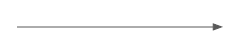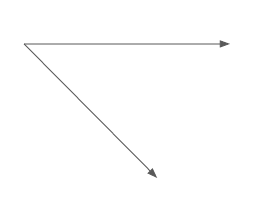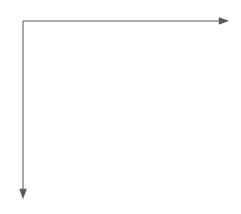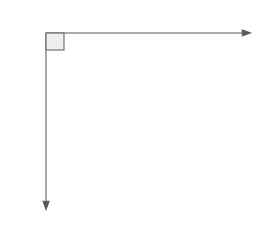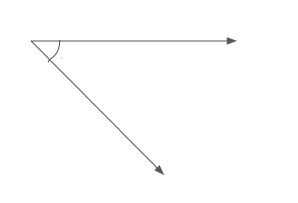Explanation:

In order to construct aangle using a ray, first recall what aangle and ray are.

An angle in the most basic terms is when two rays having the same vertex, are going in different directions.

Now, recall that aangle occurs when the lines or rays of the angle intersect perpendicularly.

Since the problem gives the starting ray,which is a horizontal ray, then to create aangle, a vertical ray needs to be constructed from the same vertex. To identify aangle it is common practice to draw a square at the vertex to show the angle measurement. This results in the final solution.### Example Question #1 : Construct Advanced Geometric Figures

Given a line, what is the first step taken to construct a parallel line?

Find the-intercept of the given line.

Identify the two lines intersection point.

Find the-intercept of the given line.

Calculate the slope of the given line.

Take the negative reciprocal of the given line's slope.

Calculate the slope of the given line.

Explanation:

To identify the first step that must be taken when constructing a parallel line, there is some information about the given line that needs to be clearly established first.

First recall what it means to be parallel. For lines to be parallel, their slopes must be equal and they must have differentandintercepts. If two lines have the same intercepts and the same slope they are not parallel lines, they are the same line. Parallel lines mean that two lines will never intersect.

From here, look at the possible answers to decide which one is best.

"Identify the two lines intersection point." by definition of parallel lines this is false since parallel lines never intersect.

"Find the-intercept of the given line." and "Find the-intercept of the given line." could possible be considered among the steps to constructing parallel lines but not necessarily.

"Take the negative reciprocal of the given line's slope." Taking this would give the slope of a perpendicular line.

"Calculate the slope of the given line." in order to construct a parallel line, the slope must first be calculated on the given line. Therefore, this is the best answer.

### Example Question #2 : Construct Advanced Geometric Figures

Given a line, what is the first step taken to construct a perpendicular line?

Take the negative reciprocal of the given line's slope.

Find the-intercept of the given line.

Either calculate the slope of the given line or identify the intersection point of the two lines.

Calculate the slope of the given line.

Identify the two lines intersection point.

Either calculate the slope of the given line or identify the intersection point of the two lines.

Explanation:

To identify the first step that must be taken when constructing a perpendicular line, there is some information about the given line that needs to be clearly established first.

First recall what it means to be perpendicular. For lines to be perpendicular, their slopes must be opposite reciprocals of each other and they must have one point of intersection.

From here, look at the possible answers to decide which one is best.

"Find the-intercept of the given line."  doesn't necessarily help when constructing a perpendicular line.

"Take the negative reciprocal of the given line's slope." Taking this would give the slope of a perpendicular line but in order to do so the slope must be known of the given line.

"Calculate the slope of the given line." in order to construct a perpendicular line, the slope must be calculated on the given line.

"Either calculate the slope of the given line or identify the intersection point of the two lines." In order to construct a perpendicular line, the intersection point must be found and the slope of the given line must be found before the slope of the perpendicular line is found. Therefore, this is the best answer.

### Example Question #2 : Construct Advanced Geometric Figures

To construct a regular pentagon, what tools are required?

Straight Edge, Compass, Pencil, Paper

Straight Edge, Compass, Pencil, Paper, Measuring Device

Straight Edge, Compass, Pencil, Measuring Device

Compass, Pencil, Paper, Measuring Device

Straight Edge, Pencil, Paper, Measuring Device

Straight Edge, Compass, Pencil, Paper, Measuring Device

Explanation:

In order to know which tools are required to construct a regular pentagon first recall the attributes of a pentagon and the possible geometric tools used to construct figures.

A regular pentagon by definition is a five sided figure. All sides of the figure are equal and every interior angle measures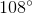.

Geometric tools used to construct figures by hand include the follow:

Paper, Pencil, Compass, Ruler, Straight Edge, Protractor, Etc.

Geometric tools used to construct figures using technology include the following:

Graphing Calculators, Computer Programs, Etc.

Since the figure in question is a regular pentagon, to construct it by hand will require the use of a compass to ensure the angles are all equaled to. Also, a straight edge is required to ensure the lines are straight and a measuring device such as a rule to ensure the side lengths are equal.

Of the possible answer choices, three list the Straight Edge, Compass, and Measuring Device. If these tools are being used then the figure is being drawn by hand therefore, pencil and paper are also required. Therefore, the correct solution is:

"Straight Edge, Compass, Pencil, Paper, Measuring Device"

### Example Question #3 : Construct Advanced Geometric Figures

Given two rays, how can a line be constructed?

Connect the vertices of the rays and have their directions pointfrom each other.

Two rays cannot be constructed into one line.

Connect the vertices of the rays and have their directions pointfrom each other.

Have their directions pointfrom each other.

Connect the vertices of the rays and have their directions pointfrom each other.

Explanation:

Recall that a ray is a line segment that has a vertex at one end and extends at the other. The extension is depicted by an arrow. In order for two rays to become one line segment the vertices must be connected and the arrows going in the opposite direction. For the rays to be going in the opposite direction they must haveangle between them.

"Connect the vertices of the rays and have their directions pointfrom each other."